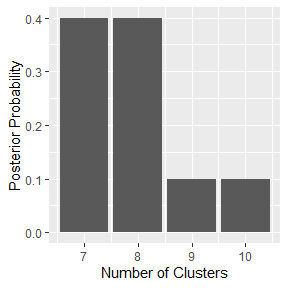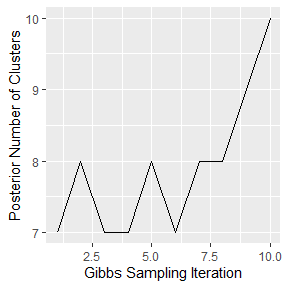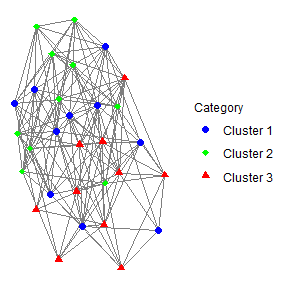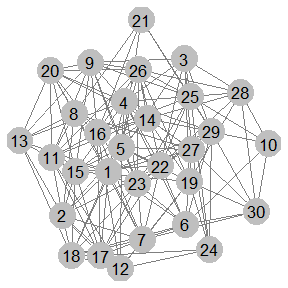# matrix-clustering-CONSTANT-vignette

library(tip)
#> Registered S3 method overwritten by 'GGally':
#>   method from
#>   +.gg   ggplot2

# A function to generate random matrices from a matrix normal distribution
random_mat_normal <- function(mu, num_rows, num_cols){
LaplacesDemon::rmatrixnorm(M = matrix(mu,
nrow = num_rows,
ncol = num_cols),
U = diag(num_rows),
V = diag(num_cols))
}

# Generate 3 clusters of matrices
p <- 5
m <- 3
c1 <- lapply(1:10, function(x) random_mat_normal(mu = 0, num_rows = m, num_cols = p))
c2 <- lapply(1:10, function(x) random_mat_normal(mu = 5, num_rows = m, num_cols = p))
c3 <- lapply(1:10, function(x) random_mat_normal(mu = -5, num_rows = m, num_cols = p))

# Put all the data into a list
data_list <- c(c1,c2,c3)

# Create a vector of true labels. True labels are only necessary
# for constructing network graphs that incorporate the true labels;
# this is often useful for research.
true_labels <- c(rep("Cluster 1", length(c1)),
rep("Cluster 2", length(c2)),
rep("Cluster 3", length(c3)))

distance_matrix <- matrix(NA,
nrow = length(true_labels),
ncol = length(true_labels))
# Distance matrix
for(i in 1:length(true_labels)){
for(j in i:length(true_labels)){
distance_matrix[i,j] <- SMFilter::FDist2(mX = data_list[[i]],
mY = data_list[[j]])
distance_matrix[j,i] <- distance_matrix[i,j]
}
}

# Compute the temperature parameter estiamte
temperature <- 1/median(distance_matrix[upper.tri(distance_matrix)])

# For each subject, compute the point estimate for the number of similar
# subjects using  univariate multiple change point detection (i.e.)
init_num_neighbors = get_cpt_neighbors(.distance_matrix = distance_matrix)
#> Warning in BINSEG(sumstat, pen = pen.value, cost_func = costfunc, minseglen
#> = minseglen, : The number of changepoints identified is Q, it is advised to
#> increase Q to make sure changepoints have not been missed.

#> Warning in BINSEG(sumstat, pen = pen.value, cost_func = costfunc, minseglen
#> = minseglen, : The number of changepoints identified is Q, it is advised to
#> increase Q to make sure changepoints have not been missed.

#> Warning in BINSEG(sumstat, pen = pen.value, cost_func = costfunc, minseglen
#> = minseglen, : The number of changepoints identified is Q, it is advised to
#> increase Q to make sure changepoints have not been missed.

#> Warning in BINSEG(sumstat, pen = pen.value, cost_func = costfunc, minseglen
#> = minseglen, : The number of changepoints identified is Q, it is advised to
#> increase Q to make sure changepoints have not been missed.

#> Warning in BINSEG(sumstat, pen = pen.value, cost_func = costfunc, minseglen
#> = minseglen, : The number of changepoints identified is Q, it is advised to
#> increase Q to make sure changepoints have not been missed.

#> Warning in BINSEG(sumstat, pen = pen.value, cost_func = costfunc, minseglen
#> = minseglen, : The number of changepoints identified is Q, it is advised to
#> increase Q to make sure changepoints have not been missed.

#> Warning in BINSEG(sumstat, pen = pen.value, cost_func = costfunc, minseglen
#> = minseglen, : The number of changepoints identified is Q, it is advised to
#> increase Q to make sure changepoints have not been missed.

#> Warning in BINSEG(sumstat, pen = pen.value, cost_func = costfunc, minseglen
#> = minseglen, : The number of changepoints identified is Q, it is advised to
#> increase Q to make sure changepoints have not been missed.

# Set the number of burn-in iterations in the Gibbs samlper
# RECOMMENDATION: burn >= 1000
burn <- 10

# Set the number of sampling iterations in the Gibbs sampler
# RECOMMENDATION: samples >= 1000
samples <- 10

# Set the subject names
names_subjects <- paste(1:dim(distance_matrix))

# Run TIP clustering using only the prior
# --> That is, the likelihood function is constant
tip1 <- tip(.data = data_list,
.burn = burn,
.samples = samples,
.similarity_matrix = exp(-1.0*temperature*distance_matrix),
.init_num_neighbors = init_num_neighbors,
.likelihood_model = "CONSTANT",
.subject_names = names_subjects,
.num_cores = 1)
#> Bayesian Clustering: Table Invitation Prior Gibbs Sampler
#> burn-in: 10
#> samples: 10
#> Likelihood Model: CONSTANT
#>
|
|                                                                      |   0%
|
|====                                                                  |   6%
|
|========                                                              |  11%
|
|============                                                          |  17%
|
|================                                                      |  22%
|
|===================                                                   |  28%
|
|=======================                                               |  33%
|
|===========================                                           |  39%
|
|===============================                                       |  44%
|
|===================================                                   |  50%
|
|=======================================                               |  56%
|
|===========================================                           |  61%
|
|===============================================                       |  67%
|
|===================================================                   |  72%
|
|======================================================                |  78%
|
|==========================================================            |  83%
|
|==============================================================        |  89%
|
|==================================================================    |  94%
|
|======================================================================| 100%
# Produce plots for the Bayesian Clustering Model
tip_plots <- plot(tip1)
# View the posterior distribution of the number of clusters
tip_plots$histogram_posterior_number_of_clusters# View the trace plot with respect to the posterior number of clusters tip_plots$trace_plot_posterior_number_of_clusters# Extract posterior cluster assignments using the Posterior Expected Adjusted Rand (PEAR) index
cluster_assignments <- mcclust::maxpear(psm = tip1@posterior_similarity_matrix)$cl # If the true labels are available, then show the cluster result via a contigency table table(data.frame(true_label = true_labels, cluster_assignment = cluster_assignments)) #> cluster_assignment #> true_label 1 2 3 4 #> Cluster 1 4 5 1 0 #> Cluster 2 7 1 1 1 #> Cluster 3 0 9 0 1 # Create the one component graph with minimum entropy partition_list <- partition_undirected_graph(.graph_matrix = tip1@posterior_similarity_matrix, .num_components = 1, .step_size = 0.001) # Associate class labels and colors for the plot class_palette_colors <- c("Cluster 1" = "blue", "Cluster 2" = 'green', "Cluster 3" = "red") # Associate class labels and shapes for the plot class_palette_shapes <- c("Cluster 1" = 19, "Cluster 2" = 18, "Cluster 3" = 17) # Visualize the posterior similarity matrix by constructing a graph plot of # the one-cluster graph. The true labels are used here (below they are not). ggnet2_network_plot(.matrix_graph = partition_list$partitioned_graph_matrix,
.subject_names = NA,
.subject_class_names = true_labels,
.class_colors = class_palette_colors,
.class_shapes = class_palette_shapes,
.node_size = 2,
#> Warning: Duplicated override.aes is ignored.# If true labels are not available, then construct a network plot
.add_node_labels = TRUE)